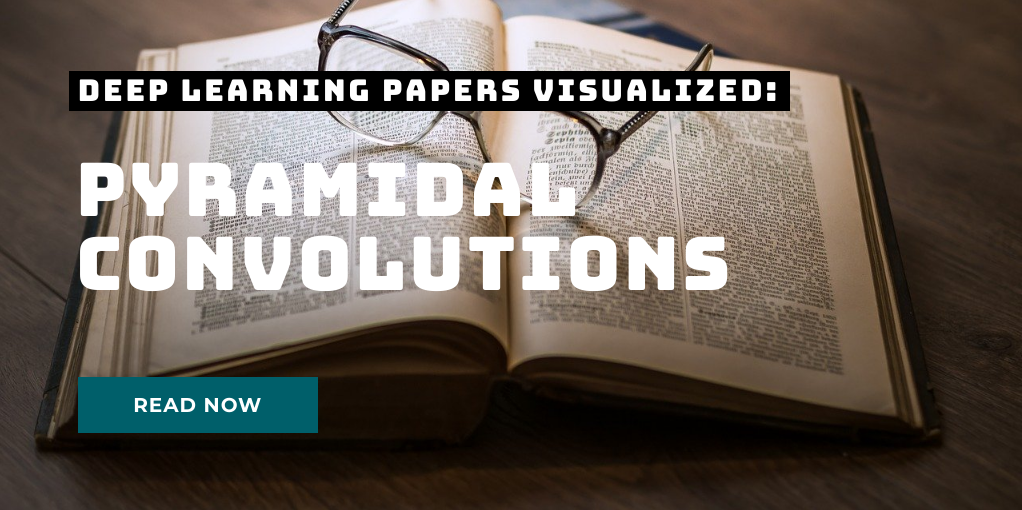# Depthwise Separable Convolutions in PyTorch

In many neural network architectures like MobileNets, depthwise separable convolutions are used instead of regular convolutions. They have been shown to yield similar performance while being much more efficient in terms of using much less parameters and less floating point operations (FLOPs). Today, we will take a look at the difference of depthwise separable convolutions to standard convolutions and will analyze where the efficiency comes from. Short recap: standard convolution In standard convolutions, we are analyzing an input map of height H and width W comprised of C channels.

# Pyramidal Convolution: Rethinking Convolutional Neural Networks for Visual RecognitionToday’s paper: Pyramidal Convolution by Duta et al. This is the third paper of the new series Deep Learning Papers visualized and it’s about using convolutions in a pyramidal style to capture information of different magnifications from an image. The authors show how a pyramidal convolution can be constructed and apply it to several problems in the visual domain. What’s really interesting is that the number of parameters can be kept the same while performance tends to improve.
Follow me on twitter!